# Retrieving the Column Header that Corresponds with a Matched Value

If you want to retrieve the column header that corresponds with a matched value,you can use a combination of INDEX, MAX, SUMPRODUCT & COLUMN functions to extract the output.

INDEX: Returns a value or reference of the cell at the intersection of a particular row and column, in a given range.

Syntax: =INDEX(array,row_num,column_num)

MAX: Returns the maximum number from a range of cells or array. For example,if a list of numbers contains 5, 6, 7, 8, 9 & 10, then the output will be 10.

Syntax =MAX(number1,number2,...)

There can be a maximum 255 arguments.Refer below shown screenshot: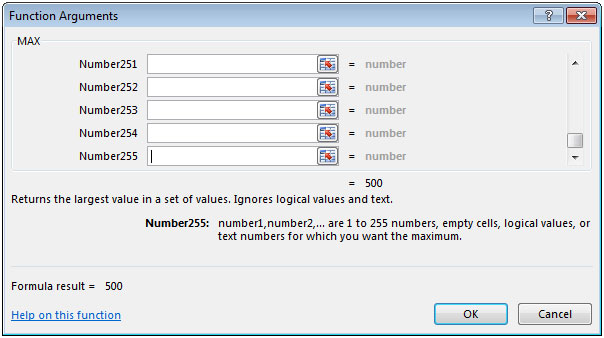SUMPRODUCT: Returns the sum of the products of corresponding ranges or arrays.

Syntax: =SUMPRODUCT(array1,array2,array3,...)

array1,array2,….. array255 are the ranges of cells or arrays that you want to multiply & then add them.

There can be maximum 255 arrays in SUMPRODUCT function.Refer below shown screenshot: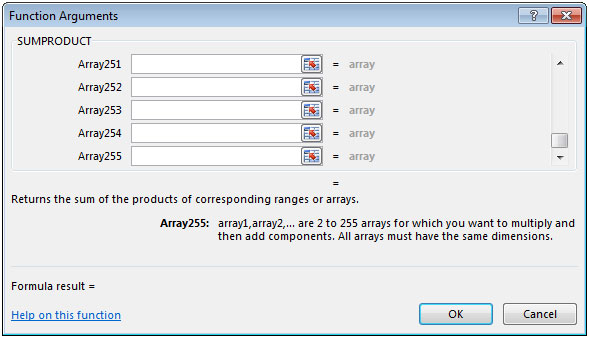COLUMN: Returns the column number of a reference.

Syntax: =COLUMN(reference)

Let us take an example:

We have 3 column headers that contain numeric values.We need a formula to lookup in cell E2 & retrieve result in cell F2 should be the heading of that column.

Selecting a value from column B in cell E2 the result should be the column heading in cell F2.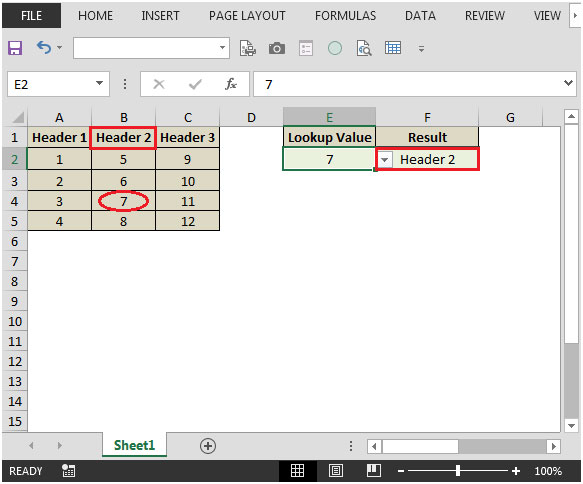lookup value is 5 refer below snapshot: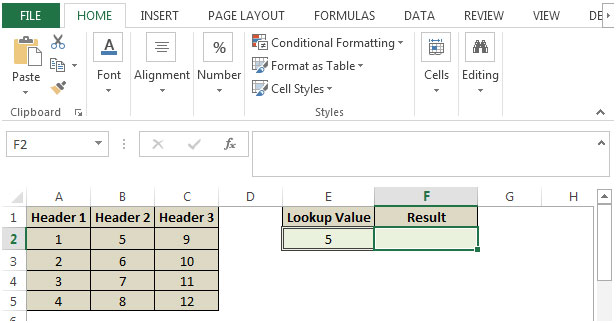• In cell F2, the formula would be
• =INDEX(\$A\$1:\$C\$1,SUMPRODUCT(MAX((\$A\$2:\$C\$5=E2)*(COLUMN(\$A\$2:\$C\$5))))-COLUMN(\$A\$1)+1)
• Press enter on your keyboard.
• The function will return the row name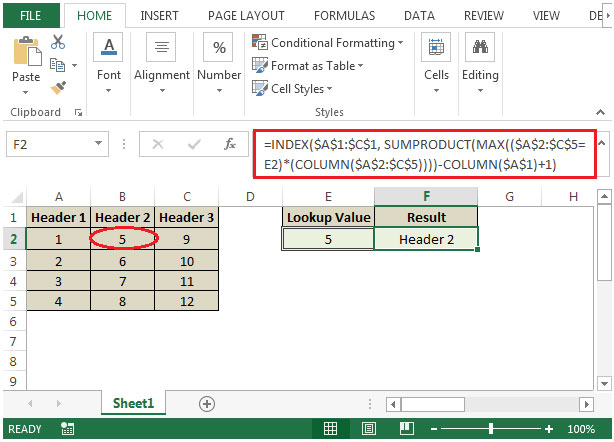• The output we get is “Header 2” because lookup 5 is found in column B.
• If we change the value in cell E2 from 5 to 10 then the formula would automatically change the result accordingly.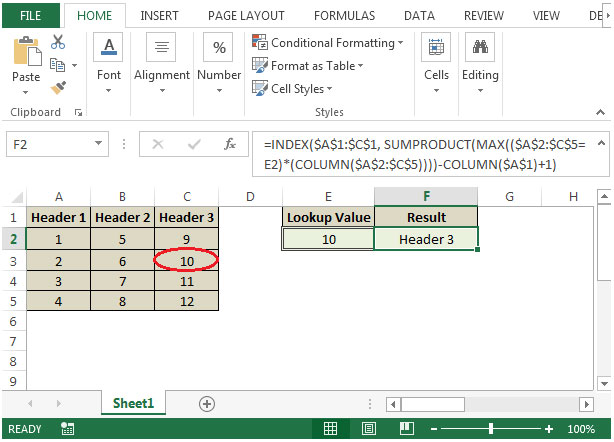1.Thank you so much. Very helpful tip.

2.This formula breaks if any of the cells in the lookup range are blank. How do I get this formula to ignore blank cells in the data range?

3.What if you have 2 of the same look up numbers can you make the results of both headers?

4.how do i make it so that the exact value is looked up and column header retrieved? I looked up a value that isn't within the table on purpose and just got the closest value -> column header of that closest value. I would like it to say error to tell me the value i'm looking for doesn't exist.

•Bingledink Humperdorf

=iferror(INDEX(\$A\$1:\$C\$1,SUMPRODUCT(MAX((\$A\$2:\$C\$5=E2)*(COLUMN(\$A\$2:\$C\$5))))-COLUMN(\$A\$1)+1),"")

Terms and Conditions of use

The applications/code on this site are distributed as is and without warranties or liability. In no event shall the owner of the copyrights, or the authors of the applications/code be liable for any loss of profit, any problems or any damage resulting from the use or evaluation of the applications/code.